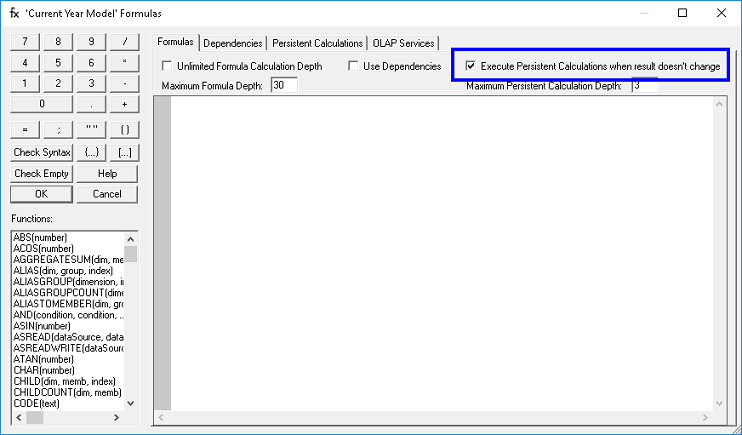Persistent Calculations – Design Mode OptionExecute Persistent Calculations when result doesn’t change option

This check box can be found in the top right section of the formula editor dialog box.Enabling this option is typically used during the development or design of persistent calculations. When this selection is disabled, a persistent calculation is only triggered when the RHS or driving variables/factors of the persistent calculation change. When this selection is enabled, the persistent calculation is executed even if the driving variable/factors remain the same and has not changed. This feature is helpful when designing and modifying persistent calculation formulas as it allows a data file of the driving variables/factors to be imported after modifying formulas and the calculation will trigger. This can be used as an alternative to regenerating dependencies after a formula modification is made, as importing the driving variable/factors and re-triggering the calculation can take less time than regenerating the persistent calculations for the entire cube. When a database is used in production, this option should be disabled.

Outlined below are the steps that must be followed in order to trigger the Persistent Calculation that is entered or modified in any cube:

1.   From the Formula Editor, go to the Persistent Calculations tab and enter the persistent calculations formula.

2.   Enable the check box Execute Persistent Calculations when result doesn’t change (refer to boxed item).3.   Click the OK button in the Formula editor.

4.   You will be prompted with a message that requires regenerate, click OK.5.   Export the cube with the new/edited persistent calculation formula.

6.   Import the cube with the new/edited persistent calculation formula.

Or, another alternative for triggering the Persistent Calculations is by doing the following: (this can replace steps 5 to 6 above)

• Export the cube with new/edited persistent calculation formula.
• Clear the cube.
• Import data to the newly cleared cube.